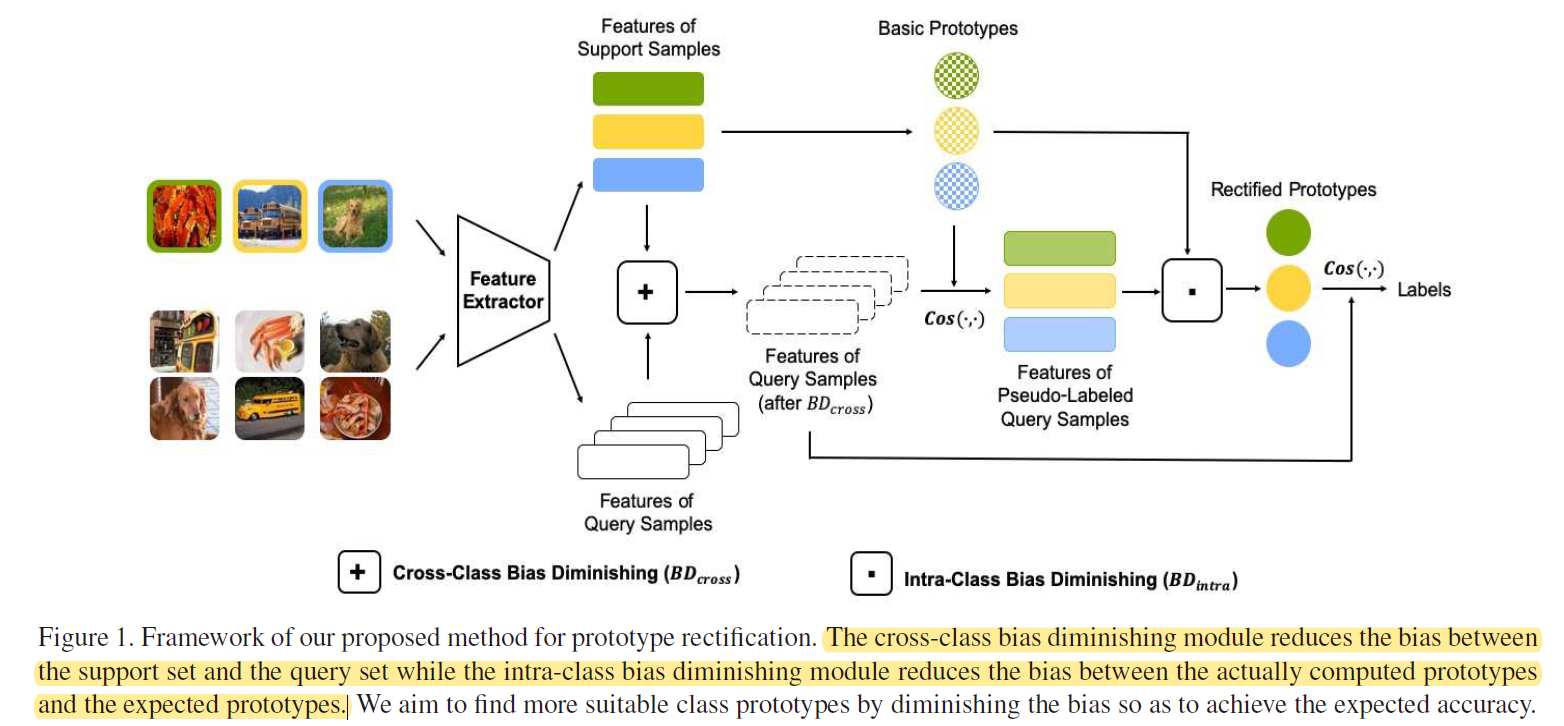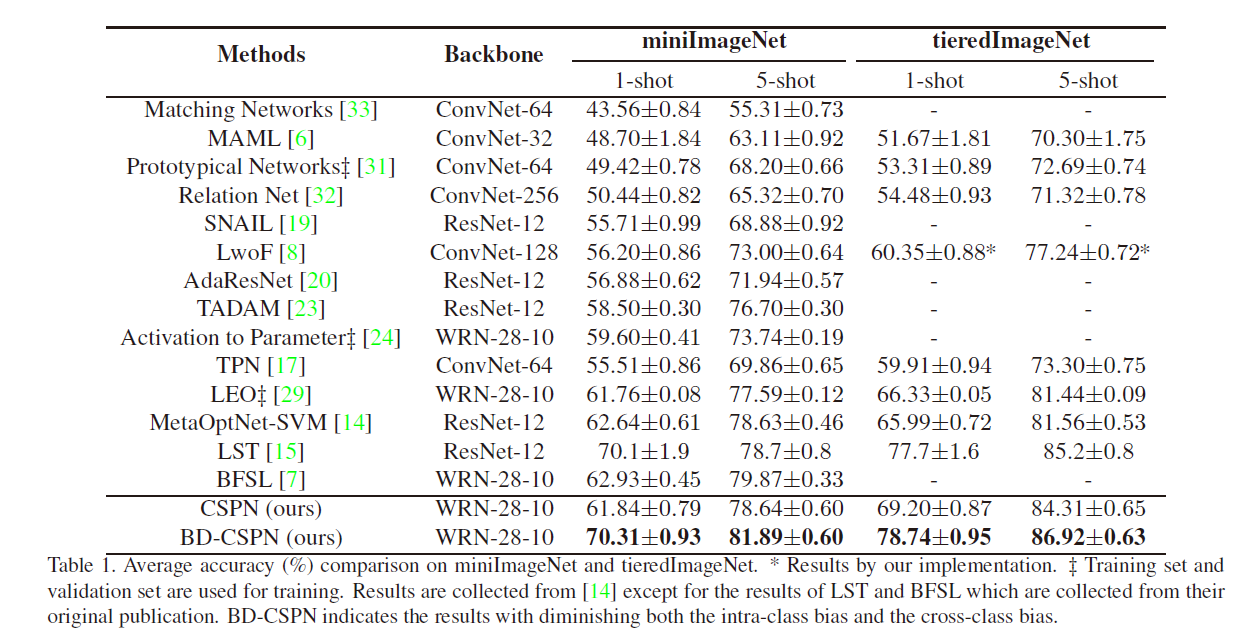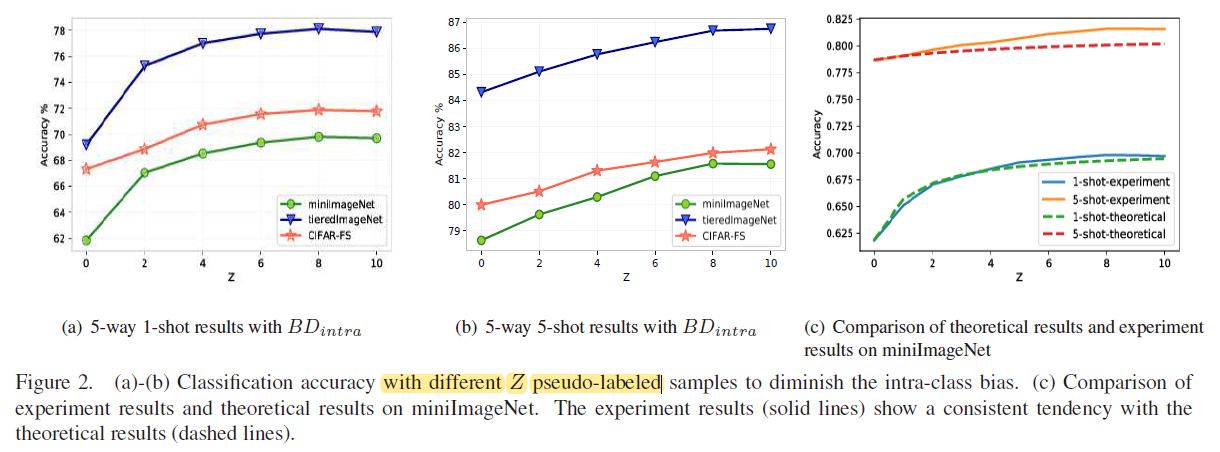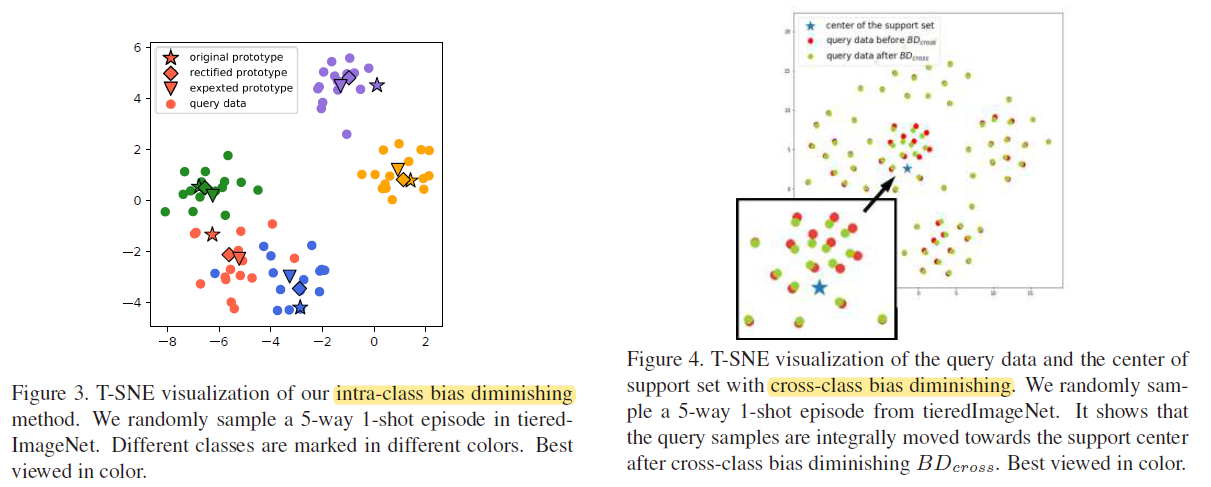# 论文阅读-Prototype Rectification for Few-Shot Learning

3 篇文章 0 订阅

pdf

ECCV 2020 Oral

## contribution

• 提出基于余弦相似度的原型网络用于计算novel类的基本原型

• bias diminishing module （BD）

• 过程

• training stage：

CSPN(cosine similarity based prototypical network),基于余弦分类器使用base类训练一个特征提取器 F θ F_\theta 以及余弦分类器 C w C_w

• inference stage：

• 使用类均值作为novel 类的基础原型，计算和样本的余弦相似度进行分类
• 基础原型和预期原型存在偏差，要消去intra-class bias和cross-class bias
• intra-class bias
• 是指类的预期无偏差原型表达与可用数据实际计算出的原型表达之间的距离。
• 通过伪标签策略把具有高置信度的没有标签的样本添加到support集合中。但是伪标签可能会带来其他误差，所以采用 weighted sum代替简单的平均
• cross-class bias：
• 是指support set和query set的代表之间的距离，通常由均值向量表示的
• 通过引入一个 ξ \xi 解决

其对于特征提取器的训练是基于整个训练集的，仅在预测过程中使用N-way K-shot的形式（也可以称之为Episode），这也意味着网络训练的参数仅仅为特征提取器的参数。两个bias消除机制作用下的prototypes产生过程没有其他需学习的参数## methodology

### CSPN

• 基于余弦分类器使用base类训练一个特征提取器 F θ ( ⋅ ) F_\theta(·) 以及余弦分类器 C ( ⋅ ∣ W ) C(·|W)

C ( F θ ( x ) ∣ W ) = Softmax ⁡ ( τ ⋅ Cos ⁡ ( F θ ( x ) , W ) ) C\left(F_{\theta}(x) \mid W\right)=\operatorname{Softmax}\left(\tau \cdot \operatorname{Cos}\left(F_{\theta}(x), W\right)\right)

• W W 是可学习的权重， τ \tau 是一个标量参数

• 损失函数： L ( θ , W ∣ D ) = E [ − log ⁡ C ( F θ ( x ) ∣ W ) ] L(\theta, W \mid \mathcal{D})=\mathbb{E}\left[-\log C\left(F_{\theta}(x) \mid W\right)\right]

• inference 阶段，在小样本上重新训练 F θ ( ⋅ ) F_\theta(·) 以及分类权重。为避免过拟合， P n P_n 的计算方式为 P n = 1 K ∑ i = 1 K X ˉ i , n P_{n}=\frac{1}{K} \sum_{i=1}^{K} \bar{X}_{i, n} ，其中 X ˉ \bar{X} 是归一化之后的support 样本

### Bias Diminishing for Prototype Rectification

#### Intra-Class Bias

• intra-class bias表达式： B intra = E X ′ ∼ p X ′ [ X ′ ] − E X ∼ p X [ X ] B_{\text {intra}}=\mathbb{E}_{X^{\prime} \sim p_{X^{\prime}}}\left[X^{\prime}\right]-\mathbb{E}_{X \sim p_{X}}[X] ，其中 p x ′ px' 是一类所有样本的分布，而 p x px 是一类可用的标记样本的分布。

• 预期的原型应该由一类中所有样本的均值特征表示。 实际上，只有一部分样本可用于训练，也就是说，几乎不可能获得预期的原型。小样本中每个类别只有K个样本，数量上比实际中所有样本数量少了很多，所以仅用K个样本计算出的原型是有偏差的。

• 采用伪标签策略来增强support集（使用query样本对support样本做了数据增强

• 使用CSPN给出预测得分，选择每个类的前top Z Z 个query样本作为support集合的扩展

• 当前support集合可表示为： S ′ = S ∪ Q pseudo Z \mathcal{S}^{\prime}=\mathcal{S} \cup \mathcal{Q}_{\text {pseudo}}^{Z}

• 但是伪标签不一定预测正确，因此简单平均可能会引起新的误差，因此采用了weighted sum的机制

P n ′ = ∑ i = 1 Z + K w i , n ⋅ X ˉ i , n ′ P_{n}^{\prime}=\sum_{i=1}^{Z+K} w_{i, n} \cdot \bar{X}_{i, n}^{\prime} w i , n = exp ⁡ ( ε ⋅ Cos ⁡ ( X i , n ′ , P n ) ) ∑ j = 1 K + Z exp ⁡ ( ε ⋅ Cos ⁡ ( X j , n ′ , P n ) ) w_{i, n}=\frac{\exp \left(\varepsilon \cdot \operatorname{Cos}\left(X_{i, n}^{\prime}, P_{n}\right)\right)}{\sum_{j=1}^{K+Z} \exp \left(\varepsilon \cdot \operatorname{Cos}\left(X_{j, n}^{\prime}, P_{n}\right)\right)}

ε \varepsilon 是一个标量参数， P n P_n 是基础原型

#### Cross-Class Bias

• 指support集中的平均样本特征与query集中的平均样本特征间存在差异

• 它源自领域适应问题，其中平均值用作一阶统计信息的类型来表示数据集，cross-class bias表达式为 B cross = E X s ∼ p S [ X s ] − E X q ∼ p Q [ X q ] B_{\text {cross}}=\mathbb{E}_{X_{s} \sim p_{\mathcal{S}}}\left[X_{s}\right]-\mathbb{E}_{X_{q} \sim p_{\mathcal{Q}}}\left[X_{q}\right]

• 对每个归一化的query 数据 X ˉ q \bar{X}_q 加上一个shifting item ξ \xi

ξ = 1 ∣ S ∣ ∑ i = 1 ∣ S ∣ X ˉ i , s − 1 ∣ Q ∣ ∑ j = 1 ∣ Q ∣ X ˉ j , q \xi=\frac{1}{|\mathcal{S}|} \sum_{i=1}^{|\mathcal{S}|} \bar{X}_{i, s}-\frac{1}{|\mathcal{Q}|} \sum_{j=1}^{|\mathcal{Q}|} \bar{X}_{j, q}

## experimentT-SNE可视化:## 反思

• 本文提出了传统的原型表达进行减小优化，减小了intra-class bias和cross-class bias两种偏差
• 和简单求平均相比，除了分类器换为余弦分类器以外，没有引入多余的训练参数

## 参考链接

https://zhuanlan.zhihu.com/p/109075199

07-071448
08-141万+08-02175
07-143584
06-193344
07-17278
04-122420
12-273万+
12-221701
10-10572
11-06241
04-012万+
11-22177
06-184453
04-23222
04-26814
12-01353
11-29386
11-30704
11-30403点击重新获取扫码支付余额充值